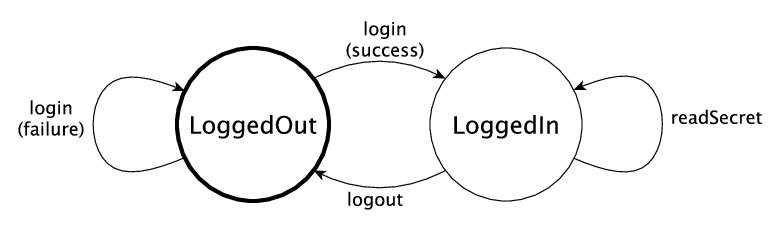# 用类型表示状态机¶## 为数据存储系统定义接口¶

```data Access = LoggedOut | LoggedIn
```

```Store : Access -> Type
```

```interface DataStore (m : Type -> Type) where
Store : Access -> Type
```

```connect : ST m Var [add (Store LoggedOut)]
disconnect : (store : Var) -> ST m () [remove store (Store LoggedOut)]
```

`connect` 的类型表明它会返回一个初始类型为 `Store LoggedOut` 的新资源。 反之，`disconnect` 则会给出一个状态为 `Store LoggedOut` 的资源并移除该资源。 我们可以通过以下（未完成的）定义更加清楚地看到 `connect` 做了什么：

```doConnect : DataStore m => ST m () []
doConnect = do st <- connect
?whatNow
```

```  m : Type -> Type
constraint : DataStore m
st : Var
--------------------------------------
whatNow : STrans m () [st ::: Store LoggedOut] (\result => [])
```

```doConnect : DataStore m => ST m () []
doConnect = do st <- connect
disconnect st
?whatNow
```

```  m : Type -> Type
constraint : DataStore m
st : Var
--------------------------------------
whatNow : STrans m () [] (\result => [])
```

```readSecret : (store : Var) -> ST m String [store ::: Store LoggedIn]
```

```badGet : DataStore m => ST m () []
badGet = do st <- connect
disconnect st
```

```When checking an application of function Control.ST.>>=:
Error in state transition:
Operation has preconditions: [st ::: Store LoggedOut]
States here are: [st ::: Store LoggedIn]
Operation has postconditions: \result => []
Required result states here are: \result => []
```

```login : (store : Var) -> ST m () [store ::: Store LoggedOut :-> Store LoggedIn] -- 类型不正确！
```

```data LoginResult = OK | BadPassword
```

```login : (store : Var) ->
ST m LoginResult [store ::: Store LoggedOut :->
(\res => Store (case res of
OK => LoggedIn
```

```logout : (store : Var) -> ST m () [store ::: Store LoggedIn :-> Store LoggedOut]
```

```interface DataStore (m : Type -> Type) where
Store : Access -> Type

connect : ST m Var [add (Store LoggedOut)]
disconnect : (store : Var) -> ST m () [remove store (Store LoggedOut)]

readSecret : (store : Var) -> ST m String [store ::: Store LoggedIn]
login : (store : Var) ->
ST m LoginResult [store ::: Store LoggedOut :->
(\res => Store (case res of
OK => LoggedIn
logout : (store : Var) -> ST m () [store ::: Store LoggedIn :-> Store LoggedOut]
```

## 用数据存储接口编写函数¶

```getData : (ConsoleIO m, DataStore m) => ST m () []
```

```getData : (ConsoleIO m, DataStore m) => ST m () []
getData = do st <- connect
?whatNow
```

```  m : Type -> Type
constraint : ConsoleIO m
constraint1 : DataStore m
st : Var
--------------------------------------
whatNow : STrans m () [st ::: Store (case ok of
OK => LoggedIn
(\result => [])
```

```getData : (ConsoleIO m, DataStore m) => ST m () []
getData = do st <- connect
case ok of
OK => ?whatNow_1
```

```--------------------------------------
whatNow_1 : STrans m () [st ::: Store LoggedIn] (\result => [])
```

```--------------------------------------
whatNow_2 : STrans m () [st ::: Store LoggedOut] (\result => [])
```

`?whatNow_1` 中，由于登录成功，因此可以读取机密数据并将它显示在终端上：

```getData : (ConsoleIO m, DataStore m) => ST m () []
getData = do st <- connect
case ok of
OK => do secret <- readSecret st
putStrLn ("Secret is: " ++ show secret)
?whatNow_1
```

```getData : (ConsoleIO m, DataStore m) => ST m () []
getData = do st <- connect
case ok of
OK => do secret <- readSecret st
putStrLn ("Secret is: " ++ show secret)
logout st
disconnect st
```

```When checking argument prf to function Control.ST.delete:
Can't find a value of type
InState st (State st) [st ::: Store LoggedOut]
```

```getData : (ConsoleIO m, DataStore m) => ST m () []
getData = do st <- connect
disconnect st
putStrLn ("Secret is: " ++ show secret)
logout st
disconnect st
```

```*Login> :exec run {m = IO} getData
When checking an application of function Control.ST.run:
Can't find implementation for DataStore IO
```

## 实现接口¶

```implementation DataStore IO where
```

```implementation DataStore IO where
Store x = ?DataStore_rhs_1
connect = ?DataStore_rhs_2
disconnect store = ?DataStore_rhs_3
logout store = ?DataStore_rhs_6
```

```Store x = State String
```

```--------------------------------------
DataStore_rhs_2 : STrans IO Var [] (\result => [result ::: State String])
```

```connect = do store <- new "Secret Data"
pure store
```

```disconnect store = delete store
```

```readSecret store = read store
```

```  store : Var
--------------------------------------
DataStore_rhs_6 : STrans IO () [store ::: State String] (\result => [store ::: State String])
```

```logout store = pure ()
```

```login store = do putStr "Enter password: "
p <- getStr
if p == "Mornington Crescent"
then pure OK
```

```implementation DataStore IO where
Store x = State String
connect = do store <- new "Secret Data"
pure store
disconnect store = delete store
p <- getStr
if p == "Mornington Crescent"
then pure OK
logout store = pure ()
```

```*Login> :exec run getData